## research papersBIOLOGICALCRYSTALLOGRAPHY
ISSN: 1399-0047
Volume 57| Part 10| October 2001| Pages 1383-1389

## How to take advantage of non-crystallographic symmetry in molecular replacement: locked' rotation and translation functions

aDepartment of Biological Sciences, Columbia University, New York, NY 10027, USA
*Correspondence e-mail: tong@como.bio.columbia.edu

(Received 26 March 2001; accepted 12 June 2001)

Many protein molecules form assemblies that obey point-group symmetry. These assemblies are often situated at general positions in the unit cell such that the point-group symmetry of the assembly becomes non-crystallographic symmetry (NCS) in the crystal. The presence of NCS places significant constraints on structure determination by the molecular-replacement method. The locked rotation and translation functions have been developed to take advantage of the presence of NCS in this structure determination, which generally requires four steps. (i) The locked self-rotation function is used to determine the orientation of the NCS assembly in the crystal, relative to a pre-defined standard' orientation of this NCS point group. (ii) The locked cross-rotation function is used to determine the orientation of one monomer of the assembly in the standard orientation. This calculation requires only the structure of the monomer as the search model. (iii) The locked translation function is used to determine the position of this monomer relative to the center of the assembly. Information obtained from steps (ii) and (iii) will produce a model of the entire assembly centered at the origin of the coordinate system. (iv) An ordinary translation function is used to determine the center of the assembly in the crystal unit cell, using as the search model the structure of the entire assembly produced in step (iii). The locked rotation and translation functions simplify the structure-determination process in the presence of NCS. Instead of searching for each monomer separately, the locked calculations search for a single rotation or translation. Moreover, the locked functions reduce the noise level in the calculation, owing to the averaging over the NCS elements, and increase the signals as all monomers of the assembly are taken into account at the same time.

### 1. Introduction

Many proteins function as macromolecular assemblies. The monomers in such assemblies are often related to each other by point-group symmetry. For example, many protein homotetramers obey 222 point-group symmetry, while the protein capsid of icosahedral viruses possesses 532 point-group symmetry. When such assemblies are crystallized, the point-group symmetry of the assembly may superimpose with the crystallographic symmetry such that the assemblies are located at special positions in the unit cell. However, it often happens that the assemblies are located at general positions in the unit cell. In such cases, the point-group symmetry of the assembly exists within the asymmetric units of the crystal and thereby the symmetry of the assembly becomes non-crystallographic symmetry (NCS) in the crystal.

Traditionally, the individual molecules of the assembly are treated separately in the molecular-replacement (MR) calculation, with no assumption of or regard to the NCS of the assembly. However, the presence of NCS introduces significant constraints on structure solution by the molecular-replacement method. A correct solution from the MR calculation must obey the NCS of the assembly. Therefore, it is more appropriate in these cases to constrain the MR calculations such that any solution that is obtained will obey the NCS of the assembly. In other words, such MR calculations are locked to the NCS of the assembly, hence the name locked rotation and locked translation functions. The concept of locked self-rotation function was first proposed in 1972, in the study of the orientations of the tetramer of glyceraldehyde 3-­phosphate dehydrogenase (Rossmann et al., 1972).

The locked rotation and translation functions offer many advantages over the traditional MR calculations. First of all, a single rotation and translation can define the entire assembly, thereby simplifying the MR calculations. Traditional methods will need to define the orientation and position of each monomer of the NCS and this becomes extremely cumbersome in cases of high symmetry. More importantly, the locked MR calculations consider the contributions of the entire assembly at the same time. This should give rise to stronger signals in the calculation, especially for cases of high NCS. Not surprisingly, the locked rotation function (RF) has found the widest application in virus crystallography, owing to the high NCS that is often involved. However, the locked MR calculations should apply to most cases of NCS point groups.

In cases where the NCS does not belong to a point group (improper symmetry), the application of the locked MR calculations becomes more difficult. For the locked RF calculations, the difficulty lies in the definition of the standard orientation of the assembly (see below). This requires knowledge of the orientations of the NCS axes relative to each other, which are generally not known beforehand with improper symmetry. In comparison, for a point group (proper symmetry) these relative orientations are fixed. In any event, if a standard orientation can be defined, the locked RF can be applied to cases where the NCS is not a point group. On the other hand, the application of the locked translation function is limited to cases where the NCS is a point group. For ease of discussion, here we will consider only cases where the NCS is a point group.

### 2. The locked self-rotation function

When the crystal contains NCS, self-rotation functions (self RFs) are used to determine the orientations of the NCS elements in the crystal unit cell. Ordinary self-RF calculations make no assumptions about the NCS and determine the orientations of the NCS elements independently of each other. However, often the nature of the NCS is known beforehand. For example, a protein that migrates as a tetramer on gel-filtration columns may form a complex that obeys 222 point-group symmetry. Similarly, icosahedral viruses are expected to have 532 symmetry. With knowledge of the possible NCS point group, the self-RF calculations can be locked to this point group, giving rise to the locked self RF (Tong & Rossmann, 1990).

Three steps are involved in the calculation of a locked self RF.

 (i) Define the standard orientation of the NCS point group. This standard orientation can be considered as a reference orientation for the NCS point group. It is usually defined such that the rotation matrices of the point group have simple forms. For example, for 222 point-group symmetry, the standard orientation can be defined such that the three twofold axes are parallel to the three Cartesian coordinate axes. With this definition, the off-diagonal elements of the rotation matrices are all zero. Additional considerations in the definition of the standard orientation are discussed in the section on the locked cross-rotation function.Once the standard orientation is defined, any arbitrary orientation of the NCS point group can be related to the standard orientation by a single rotation. Conversely, by applying different rotations to the standard orientation, any orientation of the NCS point group can be generated. Mathematically, assume [In] (n = 1, …, N) is the collection of NCS point-group rotation matrices in the standard orientation and a rotation [E] is applied to the standard orientation. This will bring the NCS point group to a new orientation and the NCS rotation matrices in this new orientation, [ρn], are given by (Tong & Rossmann, 1990)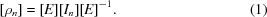(ii) Calculate the locked self-RF values for a collection of rotation angles ([E]). This collection of angles should either cover the entire unique region of the rotation space for the locked self RF (see below) or sample the region of interest in the rotation space. For each rotation [E], the ordinary self-RF value (Rn) for each of the NCS rotation matrices in the new orientation ([ρn], equation 1) is calculated. The locked self-RF value (RL) for this rotation is defined as the average of the ordinary RF values over the NCS elements (Tong & Rossmann, 1990),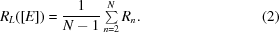Note that in the equation above the summation starts from 2, as it is assumed that [I1] is the identity matrix and therefore R1 is a constant independent of the rotation [E]. (iii) Identify the peaks in the locked self-RF map. The correct solution is expected to be one that has a high locked self-RF value. If necessary, the directions of the NCS elements for this solution can be calculated and compared with the ordinary self-rotation functions to confirm that the locked self-­RF result is correct.
The locked self RF simplifies the task of defining the orientation of an NCS assembly. Instead of searching for N − 1 peaks in the ordinary self RF, a single peak is sought in the locked self RF. It must be stressed, however, that this rotation in the locked self RF is a general rotation. For example, for the 222 point group, the rotation [E] can have any κ value (in polar angles). The locked self-RF calculation in this case cannot be limited to the κ = 180° plane, in contrast to the ordinary self RF where κ would normally be fixed at 180°. As the rotation in the locked self RF is a general one, it is generally better to carry out the calculations in Eulerian angles. This also makes it easier to define the unique region of the rotation space (see below).

Another major advantage of the locked self RF is that it reduces the noise in the calculation owing to the averaging of the ordinary RF values (2). It can be expected statistically that the noise level in the RF will be reduced by a factor of (N − 1)1/2 by the averaging process and this has been shown to be roughly correct based on actual calculations (Tong & Rossmann, 1990). Therefore, for icosahedral viruses roughly an eightfold noise reduction can be achieved with the locked self RF.

The symmetry of the locked self RF is generally rather complicated. It depends on the crystallographic symmetry and the NCS and also depends on the definition of the standard orientation. To illustrate this symmetry, the 222 point group is used here as an example. Assume that the standard orientation is defined such that the twofold axes are parallel to the Cartesian coordinate axes and that a rotation [E] is applied to this standard orientation. If [H] is a 90° rotation around the Z axis, applying the rotation [E][H] to the standard orientation should produce the same orientation of the NCS as applying the rotation [E]. This is owing to the fact that the rotation [H] only swaps the X and Y axes, but does not cause a net change to the standard orientation. Similarly, a 120° rotation around the  direction will not change the standard orientation either, as it only causes a cyclic permutation of the twofold axes. Therefore, for 222 point-group symmetry, the locked self RF appears to have at least 432 symmetry, as the collection of [H] matrices have 432 symmetry (Tong & Rossmann, 1997). The unique region of rotation space in this case can be defined to cover the regions 0–90° for all three Eulerian angles. More generally, if rotation [H] satisfies the condition [H][In][H]−1 = [Im], applying [E] and [E][H] to the standard orientation will produce the same results. These two rotations are related by the symmetry of the locked self RF. Occasionally, additional symmetry of the locked self RF can be generated by the crystallographic symmetry. In practice, the locked self-RF calculations can be rather fast. One should generally cover a large region of rotation space and then classify the resulting solutions based on the orientations of the NCS that they produce.

### 3. The locked cross-rotation function

The locked self RF has found the widest use so far in macromolecular crystallography, especially for icosahedral viruses, to determine the orientation of the NCS point-group symmetry elements in the crystal unit cell. The locked cross-rotation function (locked cross RF) and the locked translation function can be used to solve the structure of the crystal when the atomic model of only the monomer of the NCS assembly is available. For example, the structure of the monomer may have been determined in a different crystal form, by NMR or other methods, but it is not known how the monomers are arranged in the NCS assembly. Alternatively, it may be possible that the NCS assembly has undergone a reorganization, for example owing to ligand binding, leading to large changes in the relative orientation and position of the monomers in the assembly. In such a case, it is more appropriate to determine the structure of the new assembly with the model of the monomer.

With traditional MR methods, the individual monomers of the assembly are treated essentially independently in such a structure determination. The orientation and position of one monomer is determined first, followed by the determination of the second and additional monomers. This procedure is not only tedious, it also suffers from having low signals in the calculation, especially for locating the first monomer when the NCS is high. For example, with an assembly obeying 222 symmetry, the first monomer will only account for 25% of the diffracting power of the crystal and this will reduce the signals in both the ordinary RF and TF calculations to locate this monomer.

Similar to the locked self RF, one can take advantage of the presence of NCS in such a structure determination. The entire NCS assembly is considered in the locked calculations, which should increase the signal and reduce the noise. Overall, four steps are needed in the calculations that utilize the NCS (Fig. 1).

 (i) Determine the orientation of the NCS in the crystal by the locked self RF. This is discussed in the previous section and will lead to the determination of the rotation [E]. (ii) Determine a rotation [F] that relates the orientation of the monomer search model and one monomer of the NCS assembly in the standard orientation. Expansion by the NCS will then define the orientations of all the molecules of the assembly. This is the locked cross RF. (iii) Determine the position of the monomer relative to the center of the NCS by the locked translation function. Expansion by the NCS will then define the entire NCS assembly, centered (arbitrarily) at (0, 0, 0) in space. (iv) Determine the center of the NCS assembly in the crystal unit cell. A model for the entire assembly is produced in step (iii). Therefore, an ordinary TF can be used to determine the center of this assembly in the crystal.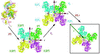Figure 1 Schematic drawing showing the various steps in a structure determination by the locked rotation and translation functions. Step (i), the locked self RF, is not shown. The locked cross RF (step ii) determines the rotation [F] and the locked translation function (step iii) determines the vector V0. This produces a model of the entire NCS assembly. Finally, an ordinary TF (step iv) determines the center of this assembly in the crystal unit cell, t.

For the locked cross RF, assume [F] is a rotation that makes the orientation of the monomer search model the same as one of the monomers of the assembly in the standard orientation; the orientations of all the monomers in the crystal unit cell is then given by (Tong & Rossmann, 1997),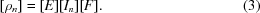In other words, [ρn] represents the (cross-) rotational relationship between the monomer search model and the monomers of the assembly in the crystal. Therefore, an ordinary cross RF value Rn can be calculated for each of the rotations [ρn] and the locked cross RF value is defined as the average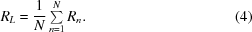Like the ordinary cross RF, the rotation [F] is completely general and can assume any value. The symmetry of the locked cross RF depends on the symmetry of the NCS point group and the definition of the standard orientation. It is however independent of the crystallographic symmetry, as the rotation [F] relates the orientation of the search model to the NCS assembly in a specific crystallographic asymmetric unit, with its orientation defined by the rotation [E]. The unique region of the rotation space for the locked cross RF can be derived from the fact that rotations [F] and [In][F] will produce the same set of rotational relationships between the search model and the crystal. Therefore, the unique region of the locked cross RF can be the same as that of an ordinary cross RF between a P1 crystal and a crystal with space-group symmetry that is equivalent to the NCS point group. For example, with a 222 tetramer, the unique region of the locked cross RF can be the same as that of an ordinary cross RF between space groups P1 and P222, which has already been defined (Rao et al., 1980). For this correspondence to work, however, the NCS standard orientation must be defined in the same way as that in the equivalent space group. For example, for 422 point-group symmetry, the standard orientation must be defined such that the fourfold axis is along the Cartesian Z axis and one of the twofold axes is along the Cartesian X axis.

The definition of the locked cross RF presented here (Tong & Rossmann, 1997) is different from the original one (Tong & Rossmann, 1990), where the rotation [F] relates the orientation of the monomer search model and a monomer of the NCS assembly in the actual orientation in the crystal. While both definitions are functionally correct, the new definition is preferred as it greatly simplifies the understanding of the symmetry of the locked cross RF.

### 4. The locked translation function

Once the orientation of one monomer of the NCS assembly is defined by the locked cross RF, the orientations of all the monomers of the assembly are defined (3). The next step in the locked MR calculation is to determine how the monomers are positioned in the NCS assembly with the locked TF (Tong, 1996). Ordinary TF calculations are based on comparisons of intermolecular vectors, where the molecules are related by the crystallographic symmetry. In contrast, the locked TF calculations are based on vectors among molecules that are related by the NCS. The locked TF does not take into account the crystallographic symmetry of the crystal.

For the locked TF, the center of the NCS assembly is placed (arbitrarily) at the origin of the coordinate system. The rotation [F] that brings the monomer search model into the same orientation as one of the monomers of the NCS assembly in the standard orientation is determined from the locked cross RF. If V0 is the translation vector that places this monomer in the same position as the monomer in the NCS assembly in the standard orientation, the entire assembly is defined by (Tong, 1996)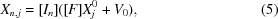where Xj0 is the atomic coordinates of the jth atom in the monomer search model. The atomic coordinates of the entire assembly in the crystal unit cell, centered at the origin, is given by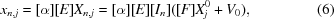where [α] is the deorthogonalization matrix (Rossmann & Blow, 1962). The calculated structure factors based on this single NCS assembly in the crystal unit cell, ignoring the crystallographic symmetry, is then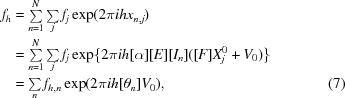where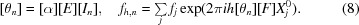The locked TF is based on the overlap between the intermolecular vectors within this NCS assembly and the observed Patterson map (Tong, 1993, 1996),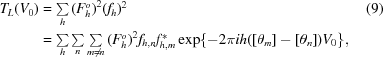where Fho is the observed structure-factor amplitude. A constant term has been omitted in (9) (Tong, 1996).

The equation for the locked TF (9) bears remarkable resemblance to that for the ordinary Patterson correlation translation function (Harada et al., 1981; Tong, 1993), with the interchange of the crystallographic (Tn) and NCS ([θn]) parameters (Tong, 1996). The evaluation of the locked TF is however more complicated. The fast Fourier transform (FFT) method cannot be applied to (9) directly, as the [θn] matrices are generally non-integral. Direct summation can be used to evaluate (9), but it would take too much time for most cases. In practice, N(N − 1)/2 FFTs of the form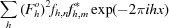are calculated first and the locked TF values are then obtained by interpolating among these transforms. In selecting solutions from the locked TF, the packing of the monomers in the NCS assembly is also examined to remove those solutions that cause serious steric clashes among the monomers.

There is no inherent symmetry in the locked TF. The unique region of the locked TF is generally a sphere or spherical shell centered at the origin of the coordinate system, if the monomer search model has been positioned such that its center is at the origin. If the monomer search model is not centered at the origin, the unique region of the locked TF will depend on both the rotation [F] and the position of the center. Therefore, the center of the monomer search model should be placed at the origin for all locked TF calculations. The radius of the sphere is determined by the distance between the center of the monomer and the center of the NCS assembly, which can be affected both by the size of the monomer and by the packing of the monomers in the assembly. Alternatively, the unique region of the locked TF can be defined as a cube centered at the origin. In special cases, the unique region of the locked TF can be limited to two dimensions. For example, if the NCS has sixfold symmetry and the standard orientation is defined such that the sixfold is along the Z axis, only the XY plane needs to be covered in the locked TF calculations.

### 5. An example

All the locked MR calculations described here are supported in the GLRF program (Tong & Rossmann, 1990, 1997), which is available freely to academic users as part of the Replace program package (Tong, 1993). To illustrate the concept and the application of the locked MR method, the structure solution of a new crystal form of the human malic enzyme is presented here as an example (Yang & Tong, 2000). Malic enzymes are tetrameric in solution and the tetramers obey 222 point-group symmetry (Bhargava et al., 1999). The tetramer interface undergoes large reorganizations depending on whether transition-state analog inhibitors are bound to the enzyme (Xu et al., 1999; Yang et al., 2000). For the example here, the monomer of the enzyme was used as the search model to solve the structure of the enzyme in a different crystal form. This new crystal belongs to the space group P21, with a tetramer of the enzyme in the asymmetric unit (Bhargava et al. , 1999).

The first step in a locked MR calculation is to determine the orientation of the NCS axes. For this example, the ordinary self RF clearly showed the orientations of the NCS twofold axes, demonstrating the 222 symmetry of the tetramer (Bhargava et al., 1999). For the locked self RF, the standard orientation of the point group was defined such that the three twofold axes are parallel to the Cartesian coordinate axes. The calculation covered the region 0–90° for each Eulerian angle with a grid interval of 3°. An ordinary self RF was calculated first with the fast rotation function (Crowther, 1972) using reflection data between 10 and 3.5 Å resolution. The radius of integration was 35 Å. The locked self-RF values were then obtained by interpolating in the ordinary self-RF map. The entire calculation took roughly 4 min of CPU time on an SGI O2 R10000 workstation.

The highest peak in the locked self RF stands out from the rest of the peaks, suggesting that it is likely to be the correct solution (Table 1). However, peaks 2–4 also have reasonably high locked self-RF values (Table 1). The orientations of the NCS elements corresponding to each of the top four peaks in the locked self-RF map were then plotted in a stereographic projection and compared with the ordinary self RF (Fig. 2). It clearly shows that the top peak in the locked self RF is the correct solution. However, peaks 2–4 are erroneous, owing to accidental overlap of one of the twofold axes with the correct orientation. Such noises in the locked self RF are expected to be more serious when the NCS is low. When the NCS is high, for example for icosahedral viruses, the background noise is reduced significantly by the averaging and the correct solution is essentially the only peak in the locked self RF (Tong & Rossmann, 1990). In addition, once two non-collinear NCS axes are matched by a rotation, the entire NCS point group is matched. Therefore, when the NCS is low it may be important to cross check the solution from the locked self RF with the results from the ordinary self RF.

 Table 1Summary of locked MR calculation with malic enzyme
 (a) Results from locked self RF to define the orientation of the NCS point group.
Peak No. θ1 θ2 θ3 Height Height/σ
1 33 33 24 488.7 13.9
2 81 75 30 430.0 10.2
3 33 33 60 422.8 9.7
4 75 78 33 400.0 8.2
5 6 18 49 377.8 6.8
 (b) Results from locked cross RF to define the orientation of the monomer model.
Peak No. θ1 θ2 θ3 Height Height/σ
1 93 87 159 1000 10.1
2 165 75 0 589.6 5.8
 (c) Results from locked TF to define the position of the monomer in the NCS assembly.
Peak No. X Y Z Height Height/σ
1 27 −2 26 1000 7.8
2 −26 −2 26 530.0 4.1
 (d) Results from ordinary TF to define the center of the NCS assembly in the crystal.
Peak No. x y z Height Height/σ CC R Contact
1 0.1389 0 0.3688 100 12.6 47.9 39.2 1
2 0.1019 0 0.0000 24.2 3.1 36.0 43.7 23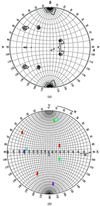Figure 2 (a) Stereographic projection showing the ordinary self RF of human malic enzyme. Reflection data between 10 and 3.5 Å resolution were used in the calculation with the program GLRF (Tong & Rossmann, 1990). The radius of integration was 35 Å. (b) Stereographic projection showing the orientation of the NCS twofold axes based on the top peak (red symbols) and the next three peaks (green, purple and cyan symbols, respectively) in the locked self RF. The top peak is the correct solution and the next three peaks arise from accidental overlap with one of the twofold axes of the NCS.

With the knowledge that the top peak in the locked self RF is correct, a fine search was then carried out using 1° intervals around the rotational parameters of this top peak. This produced more accurate parameters for the rotation [E], at 32, 34, 24°.

The locked cross RF was then calculated to determine the orientation of the monomer in the NCS assembly. As with the ordinary cross RF, the monomer search model was placed in a large P1 cell with dimensions of a = b = c = 100 Å and structure factors to 3.5 Å resolution were calculated for this artificial crystal. An ordinary cross RF was calculated with the fast RF (Crowther, 1972), using reflection data between 10 and 3.5 Å resolution and a radius of integration of 35 Å; the locked cross RF values were obtained by interpolating in this map. The entire calculation took roughly 6 min CPU time, covering the region 0–180° in θ1 and θ3, and 0–90° in θ2, with 3° grid intervals. The locked cross RF contained one significant peak whose height was about twice that of the second peak in the function (Table 1). This clearly demonstrated that the correct orientation of the monomer has been found. More accurate parameters for the rotation were obtained from a subsequent fine search, with 1° intervals in the three Euler angles.

With the knowledge of the orientation of the NCS assembly (rotation [E]) and the orientation of the monomer in this assembly (rotation [F]), the locked TF was then calculated to determine the position of this monomer relative to the center of the NCS. Reflection data between 10 and 3.5 Å resolution were used in the calculation. The monomer search model was centered at the origin of the coordinate system. The search region was defined as a spherical shell with an inside radius of 15 Å and an outside radius of 40 Å, as it is known from the structures of the other tetramers of this enzyme that the center of the monomer is about 35 Å from the center of the tetramer. The grid interval along the three axes was 1 Å. The calculation took about 6 min CPU time. There was only one significant peak in the locked TF (Table 1) and placing the monomer at this position also gives rise to reasonable packing of the monomers in the NCS assembly. Therefore, this is likely the correct solution from the locked TF. A fine search was then carried out using 0.5 Å intervals to obtain more accurate parameters for the position of the monomer.

At the completion of the locked TF calculation, the GLRF program outputs the atomic model for the entire NCS assembly in the standard orientation and centered at the origin. This model for the NCS assembly was then used in an ordinary TF calculation to determine the center of the NCS assembly in the crystal. The TF program of the Replace package was used in this example (Tong, 1993), using reflection data between 10 and 4 Å resolution. It clearly revealed the location of the NCS assembly in the crystal (Table 1). The top peak has significantly better correlation coefficient (CC) and R-factor values. In addition, there are few steric clashes among crystallographically related molecules based on this solution (Tong, 1993). This confirms that the locked MR calculations successfully determined the structure of this new crystal form of human malic enzyme.

### Acknowledgements

This research is supported by a grant from the National Science Foundation (DBI-98-76668).

### ReferencesBIOLOGICALCRYSTALLOGRAPHY